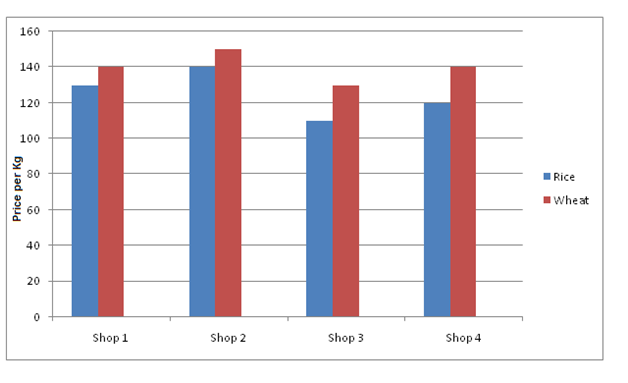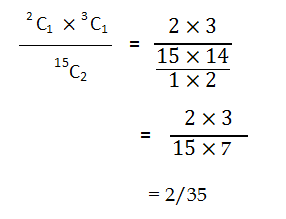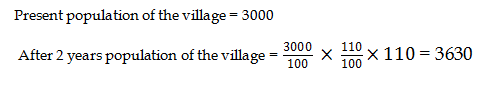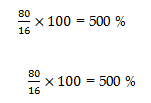# RRB ALP 2018 Practice Test Papers | Arithmetic Questions (Day-80)

Dear Aspirants, Here we have given the Important RRB ALP & Technicians Exam 2018 Practice Test Papers. Candidates those who are preparing for RRB ALP 2018 can practice these Arithmetic Questions to get more confidence to Crack RRB 2018 Examination.

[WpProQuiz 3133]

Click “Start Quiz” to attend these Questions and view Solutions

Direction (1 – 5): The given bar diagram represents the Price per kg of Rice and Wheat in Various shop of a city as follows1) A man bought 1kg of rice and 2 kg of wheat in Shop 3. How much total amount he has to pay?

a) 450

b) 540

c) 370

d) 360

2) Amir went to Shop 2 for rice purchase and if he has Rs. 500, then how many kilograms of rice he can buy?

a) 1

b) 2

c) 3

d) 4

3) What is the Average price of rice in all the shops?

a) 370

b) 137.50

c) 150

d) 200

4) What is the ratio between Total price of wheat and rice in all the shops?

a) 48: 23

b) 45: 37

c) 55: 58

d) 65: 98

5) The price of wheat in shop 1 is what percentage that of rice in shop 2?

a) 100%

b) 150%

c) 120%

d) 75%

6) A bag consists of 3 green balls, 5 yellow balls and 2 white balls. One ball is picked up randomly what is the probability of the ball is yellow colour?

a) 2/15

b) 1/5

c) 1/2

d) 3/5

7) In a bag consists of 3 green balls, 10 yellow balls and 2 white balls. Two ball is picked up randomly what is the probability of one ball is white and one ball is green?

a) 1/ 35

b) 2 / 35

c) 1 / 14

d) 2/ 17

8) In a village, population is 3000; every year population is increased by 10 %. What is the population the village after 2 years?

a) 3690

b) 3900

c) 3630

d) 3500

9) Find the value of x

78 + 50% of 68 + 657 = x + 15 × 29

a) 356

b) 334

c) 345

d) 335

10) 80 is what percentage of 16?

a) 100

b) 400

c) 500

d) 300

(110*1) + (130 * 2) = 110 + 260 = 370

1 Kg of rice in shop 2 = Rs. 140

500/140 ≈ 4

He could buy 4 kg of rice in shop 2

Average Price of Rice = (130 + 140 + 110 + 120)/4 = 137.50

Total price of Rice = 130 + 140 + 110 + 120 = 500
Total Price of Wheat = 140 + 150 + 130 + 140 = 560
500 : 560
25 : 28

(140*100)/140 = 100 %

5C1   / 10C1 = 5/10 = 1/278 + 50% of 68 + 657 = x + 15 × 29

769 = x + 435

x = 334